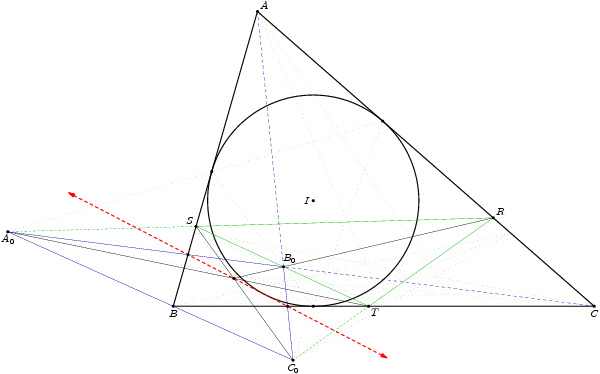### Rendering diagrams from a mathematics competition in an IPython notebook¶

We load the following IPython asymptote magics remotely.

In :
# Install the IPython magic extension
%install_ext http://raw.github.com/azjps/ipython-asymptote/master/asymptote.py

Installed asymptote.py. To use it, type:


For ease, we use two asymptote modules written by users of the forum Art of Problem Solving: cse5 and olympiad. The first abbreviates some common commands in a more functional style and the second offers a few useful geometry functions. Since they are not underneath version control to my knowledge, I have included them in the github project ipython-asymptote/examples.

### PUMaC Power Round Solution Round 2013¶

The following problems are taken from the 2011 Power Round of the Princeton University Math Competition, a week-long open-resources round of a high school mathematics competition.

General Problem Setting We are given a scalene triangle $ABC$ in plane, with incircle $\Gamma$, and we let $D$, $E$, $F$ be the tangency points of $\Gamma$ with the sides $BC$, $CA$, and $AB$, respectively. Furthermore, let $P$ be an arbitrary point in the interior of the triangle $ABC$ and let $A_{1}B_{1}C_{1}$ be its cevian triangle (that is, $A_{1}$, $B_{1}$, $C_{1}$ are the intersections of the lines $AP$, $BP$, $CP$ with the sides $BC$, $CA$, and $AB$, respectively); from $A_{1}$, $B_{1}$, $C_{1}$ draw the tangents to $\Gamma$ that are different from the triangle's sides $BC$, $CA$, $AB$, and let their tangency points with the incircle be $X$, $Y$, and $Z$, respectively.

Setting up the IPython notebook For the remainder of this notebook, we'd like to use a common set of settings, such as imports, diagram size, and pre-defined pens. From the above problem description we can also define the points that will be needed for drawing diagrams. We save these to the file pumac_power_round_2011_config.asy as follows:

In :
%%asy --root pumac_power_round_2011_config
import cse5;
size(100);

/* Setup for cse5 default pens */
pointpen = black;
pathpen = linewidth(1.5);
dotfactor = 7;

/* Some other common pens to use */
pen darkgreen = linewidth(1.5),
darkred = linewidth(1) + red,
darkblue = linewidth(1) + rgb(0, 0, 0.7),
faded = linewidth(1) + linetype("0 4"),
dots = linewidth(1)+ dotted;

/* Define points and paths described in the general problem setting */
pair B = (0,0), C = (10,0), A = (2,7);
path t = A--B--C--cycle, incirc = incircle(A,B,C);
pair I = incenter(A,B,C), D = foot(I,B,C), E = foot(I,C,A), F = foot(I,A,B), P = (4.6,1);
pair A1 = extension(B,C,A,P), B1 = extension(A,C,B,P), C1 = extension(A,B,C,P);
pair X1 = reflect(I,A1)*D, Y1 = reflect(I,B1)*E, Z1 = reflect(I,C1)*F;
pair P1 = extension(A,X1,B,Y1), V = extension(A1,X1,A,C), A2 = extension(A,X1,B,C);
pair A0 = extension(Y1,Z1,E,F), B0 = extension(X1,Z1,D,F), C0 = extension(X1,Y1,D,E);
pair R = (1.5*A + 3.5*C)/5, S = extension(A0,R,A,B), T = extension(C0,R,B,C);
pair P2 = extension(A0,T,B0,R), M = extension(A,B,A0,C), N = extension(A,C0,B,C);

/* Draw a couple of the common paths */
D(A--B--C--cycle); D(incircle(A,B,C));

/* Label some of the common points */
D(MP("A", A, NE));
D(MP("B", B));
D(MP("C", C));
D(MP("I", I, W));


Out: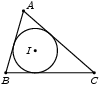1) (a) Extend $A_{1}X$ to intersect $AC$ at $V$. Show that the lines $AA_{1}$, $BV$, and $DE$ are concurrent.

(b) Let $A_{2}$ be the intersection point of $AX$ with the sideline $BC$. Show that $$\frac{A_2B}{A_2C} = \frac{s-c}{s-b} \cdot \frac{A_1B^2}{A_1C^2},$$ where $s = \frac{a + b + c}{2}$ is the semiperimeter of $\triangle ABC$. [Hint: Menelaus's Theorem may come in handy.]

(c) Prove that lines $AX, BY, CZ$ are concurrent.

In :
%%asy pumac_power_round_2011_config.asy
size(500);

/* Draw lines */
D(A--A1--X1, darkgreen);
D(B--B1--Y1, darkgreen);
D(C--C1--Z1, darkgreen);
D(A--P1--B, darkred);
D(C--Z1, darkred);
D(X1--V);
D(P1--A2, darkred);

/* Label points */
D(MP("P",P,dir(60)));
D(MP("D",D)); D(MP("E",E,NE)); D(MP("F",F,NW));
D(MP("X",X1,ENE)); D(MP("Y",Y1,1.4*dir(150))); D(MP("Z",Z1,NE)); D(P1);
D(MP("A_1",A1)); D(MP("B_1",B1,NE)); D(MP("C_1",C1,NW));
D(MP("V",V,NE)); D(MP("A_2",A2));


Out: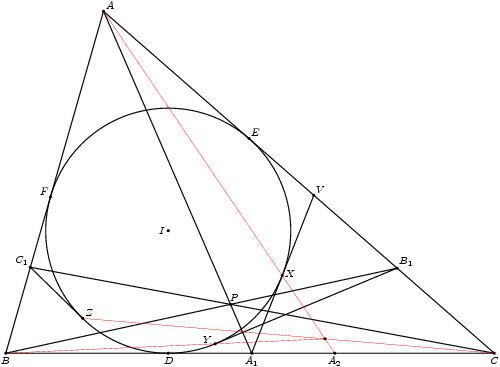2) Show that the lines $B_1C_1$, $EF$, $YZ$ concur at a point, say $A_{0}$. Similarly, the lines $C_1A_1$, $FD$, $ZX$ and $A_1B_1$, $DE$, $XY$ concur at points $B_{0}$ and $C_{0}$, respectively. [Hint: Pascal's theorem might be useful here.]

In :
%%asy pumac_power_round_2011_config.asy
size(600);

/* Draw lines */
D(Y1--A0--E); D(F--D); D(A1--C1); D(B1--C0--E);
D(D--E--F--cycle,rgb(0,0.7,0)+linewidth(1));
D(A0--B0--C0--cycle, darkblue);
D(A--B0--C, darkblue+linetype("3 3"));

/* Label points */
D(MP("D",D,SSE)); D(MP("E",E,NE)); D(MP("F",F,NW));
D(MP("X",X1,dir(-10))); D(MP("Y",Y1,(0,-2.2))); D(MP("Z",Z1,dir(220))); D(MP("P",P,dir(60)));
D(MP("A_1",A1)); D(MP("B_1",B1,NE)); D(MP("C_1",C1,NW));
D(MP("A_0",A0)); D(MP("B_0",B0,0.8*dir(30))); D(MP("C_0",C0));


Out: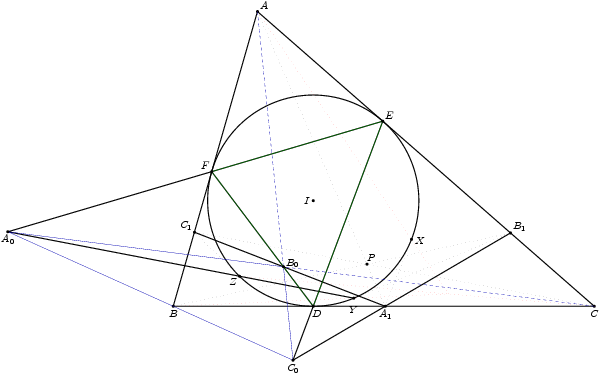3) Let $R$ a point on $AC$ and let $S$, $T$ be the intersections of $RA_{0}$, $RC_{0}$ with $AB$ and $BC$ respectively. Then, $B_{0}$ lies on $ST$.

In :
%%asy pumac_power_round_2011_config.asy
size(600);

/* Draw lines */
D(A0--B0--C0, darkblue);
D(A--B0--C, darkblue);
D(A0--R--C0, rgb(0,0.7,0)+linewidth(1));
D(A0--S, rgb(0,0.7,0)+linewidth(1)+linetype("3 3"));
D(C0--T, rgb(0,0.7,0)+linewidth(1)+linetype("3 3"));
D(S+.6*(S-T)--T+.6*(T-S), rgb(0,0.7,0)+linewidth(1)+linetype("3 3"));

/* Label points */
D(MP("D",D,SSE)); D(MP("E",E,NE)); D(MP("F",F,NW));
D(MP("A_0",A0)); D(MP("B_0",B0,dir(225))); D(MP("C_0",C0));
D(MP("R",R,NE)); D(MP("S",S,1.2*dir(215))); D(MP("T",T,(0,-1.2)));


Out: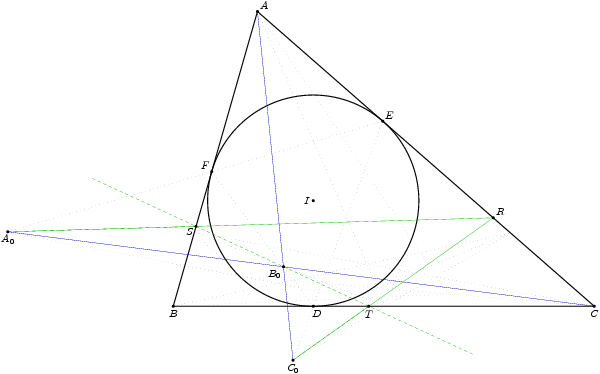4) We say that two triangles $\triangle ABC, \triangle DEF$ are perspective iff $AD, BE, CF$ are concurrent. The point of concurrency is called their perspector. Show the following pairs of triangles are perspective:

(a) $\triangle A_0B_0C_0$ and $\triangle DEF$. Their perspector lies on the incircle of $\triangle ABC$.

(b) $\triangle ABC$ and $\triangle TRS$

(c) $\triangle A_0B_0C_0$ and $\triangle TRS$. The locus of the perspector as $R$ varies along the line $AC$ is a line tangent to the incircle of $\triangle ABC$.

In :
%%asy pumac_power_round_2011_config.asy
size(600);

/* Draw lines */
D(A0--B0--C0--cycle, darkblue); D(A--B0--C, darkblue+linetype("3 3"));
D(R--S--T--cycle, rgb(0,0.7,0)+linewidth(1)); D(A0--S, rgb(0,0.7,0)+linewidth(0.7)+linetype("3 3"));
D(C0--T, rgb(0,0.7,0)+linewidth(0.7)+linetype("3 3"));
D(A0--T,linewidth(1)); D(R--P2,linewidth(1)); D(C0--S,linewidth(1));
D(M+1.2*(M-N)--N+(N-M), linewidth(1.5) + linetype("2 2") + rgb(1,0,0), Arrows(6));